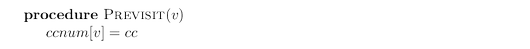# Glossary

## Algo: Connected graph

An undirected graph is connected if there is a path between any pair of vertices. The graph of the following figure is not connected because, for instance, there is no path from $A$ to $K$. However, it does have three disjoint connected regions, corresponding to the following sets of vertices: $$\{A, B, E, I, J\}\, \{C, D, G, H, K, L\}\, \{F\}\, .$$These regions are called connected components: each of them is a subgraph that is internally connected but has no edges to the remaining vertices.

Depth-first search can be used to check if a graph is connected and, more generally, to assign each node $v$ an integer ${\tt ccnum}[v]$ identifying the connected component to which it belongs. When ${\tt explore}$ is started at a particular vertex, it identifies precisely the connected component containing that vertex. And each time the DFS outer loop calls explore, a new connected component is picked out. Thus, all it takes iswhere ${\tt cc}$ needs to be initialized to zero and to be incremented each time the DFS procedure calls ${\tt explore}$.